### Inferential Statistics

We have all heard the phrase ‘statistical tests’ – for example in a newspaper report that claims ‘statistical tests show that women are better at reading maps than men’. If we wanted to know if women are better at reading maps than men we could not possibly test all the men and all the women in the world, so we just test a small group of men and a small group of women. If we find the sample of women are indeed better with maps than the sample of men, then we infer that the same is true for all men and all women. However, it isn’t quite as simple as that because we can only make such inferences using statistical (or inferential) tests. All statistical tests though are based on the idea of probability. So, before we start to look at the different statistical tests, we need to understand the role that probability plays in statistical testing as no test to guarantee human behaviour 100%.

Probability and Significance

We are all familiar with the notion of probability because we use it every day in the judgements we make about different situations.

Inferential statistics allow psychologists to make conclusions based on the probability that a particular pattern of results could have arisen by chance. If a study that found a sample of women were better at map reading than a sample of men had only arisen due to chance factors (i.e. how it happened to go on the day) rather than because a genuinely noticeable effect does exist, then it would not be correct to conclude women are better map readers than men. However, if it could not have arisen by chance or if it is extremely unlikely to have arisen by chance (e.g. because the difference between men and women was so large), then the pattern is described as significant.

Judging whether an effect is significant or not cannot be done just by looking at averages or other forms of descriptive analysis. Instead, inferential statistical tests must be carried out to ascertain whether results are significant (i.e. whether they are likely to have been down to chance or not).

‘Chance’ and ‘Significance Level’

By ‘chance’, we simply mean a probability that we will ‘risk’. You cannot be absolutely certain that an observed effect was definitely not down to chance no matter how strong the effect seems to be. However, you can state how certain you are. In general, psychologists use a probability (‘p’) ≤ 0.05, which means that there is less than or equal to a 5% probability the results did occur by chance. In other words, p ≤ 0.05 means there is at least a 5% probability that the results occurred even if there was no real effect present.

For example: Lets say that a psychologist wanted to investigate the effects of music on memory. They give their participants a memory test to complete without music and then a memory test to complete with music. The psychologist is hoping to find that the music (IV) will significantly decrease memory performance (the DV).

When carrying out the inferential test the psychologist is aware that there is no way that they can be 100% certain and conclude that the IV (music) will be the only variable to have an effect on the DV (memory) as there are many other factors that could have caused the music to have impaired participant memory (e.g. the style of music, the time of day, temperature of the room etc…) Although a psychologist will try to control for as many of these variables as they can, they can’t be 100% sure that they have controlled for everything. Iit’s like a Dr, they may carry out a blood test looking for diabetes, they may confirm that they are 99.9% sure that their patient doesn’t have diabetes, but they would rarely confirm that they are 100% sure that they don’t have diabetes as there is also a small possibility that the results are down to chance – i.e. the test may have been carried out in error at the lab etc…).

As a result, whenever a psychologist is carrying out research which leads to inferential testing, the psychologists has to make a decision how much of their findings they want to attribute to chance. As stated above, in most cases, psychologists use a probability value of 5%, this means that when drawing conclusions for a study the psychologist can report that they are 95% sure that the chance in the DV was down to the manipulation of the IV (so in the case of the example above, the psychologist would be 95% sure that it was the music that impaired participant memory performance, however, they are aware that there is a 5% probability that the change in the DV was as aresult of something other than the manipulation of the DV – A CHANCE FACTOR).

In some studies, psychologists want to be more certain – such as when they are conducting a replication of previous research or considering the effects of a new drug on health (because here in particular we would want to be very careful about taking chances). In these situations, researchers use a more stringent probability such as p ≤ 0.01, (here the psychologist would be 99% sure the IV had caused an effect in the DV but would attribute 1% that the change in the DV was down to another chance factor). In other situations, a more lenient level such as             p ≤ 0.10 might be used, such as when conducting research into a new topic. This chosen value of ‘p’ is called the significance level.

Probability values often used in research

P<0.01 (1% attributed to chance) – used when researchers are sure that the IV will have an effect on the DV (usually when a psychologist is replicating previous research where consistent findings have been obtained). This may also be used when psychologists are carrying out research in which the results need to be more or less guaranteed (e.g. when testing the effectiveness of a new drug).

P<0.05 (5% attributed to chance) – used in most pieces of research.

P<0.10 (10% attributed to chance) – used mainly when research hasn’t been carried out before.

It is good practice in psychology that once a piece of research has been found to be significant at a higher level of chance (e.g. 10%) the research is then repeated under a lower level of chance (5%). The lower the value of chance, the more striking the psychological results.

### Type 1 and Type 2 Errors

Although different levels of significance are used by psychologists, in general, most research does use p ≤ 0.05. There are good reasons for using this 5% level.

(1) Type 1 Error: If you use a level of significance that is too high (lenient), such as 10%             (or p ≤ 0.10), then you may reject a null hypothesis that is true.

Consider this example… imagine someone takes a pregnancy test and it is positive, leading the person to accept the hypothesis that they are pregnant (thus rejecting the null hypothesis that they are not pregnant). What if the test was wrong and it was a false positive? This is called a Type 1 error – rejecting a null hypothesis that is true. In research, the likelihood of a type 1 error is increased if the significance level is too high (lenient) such as 10% (i.e. there is more probability that the results were down to chance and not the effects of the IV on the DV).

(2) Type 2 Error: A type 2 error is when a person is being too stringent/harsh. A psychologists may reject the experimental hypothesis and accept the null hypothesis when in actual fact they should be accepting the experimental hypothesis and  rejecting the null hypothesis.

For example: If the result on the pregnancy test was negative even though the person was actually pregnant, the person would accept the null hypothesis that they are not pregnant when they are. This is a Type 2 error – accepting a null hypothesis that is in fact not true. In research, the likelihood of a Type 2 error is increased if the significance level is too low (stringent) such as 1%.### Using Inferential Statistical Tests

Once you know about the level of significance you are going to use, you can decide which statistical test you are going to use to analyse your data to assess whether your findings are significant or not. Different statistical tests are used for different research methods, experimental designs and levels of measurement. There are seven statistical tests that you need to know about:

Non-Parametric Tests:

1. Spearman’s Rho (or Rank)

2. Wilcoxon T

3. Mann-Whitney U

4. Chi-squared (or x2)

Parametric Tests:

5. Unrelated T-Test

6. Related T -Tests

7. Perason’s R

How do you choose which test to use?

This is simply based on the answers to 3 questions…

(1) Do I want to investigate differences or relationships? If your study was an experiment then you will be investigating differences. If your study was a correlation then you will be investigating relationships.

(2) What sort of data (or levels of measurement) do I have? (e.g. Nominal, Ordinal, Interval or Ratio).

There are several kinds of Quantitative data (or LEVELS OF MEASUREMENT) that you need to be aware of when deciding what statistical test to use in order to assess the significance of your data:-

Levels of Measurement (or Levels of Data):

(A) NOMINAL DATA: The researcher identifies categories of objects or behaviours and counts how many instances there are in each category, e.g., categories could be “conforms” and “does not conform”, where the researcher would just count how many people fell into each category – they could produce a tally under each category. This is the simplest kind of quantitative data.

(B) ORDINAL DATA If data can be placed in rank order on a scale then it is ordinal data. Positions in a race provide an example of ordinal data. The runners are ranked according to their finishing positions- 1st, 2nd, 3rd.This data does not measure how far apart the items on the scale are since they are arbitrary. For example, they do not state the time difference between runners.

Any arbitrary scale is an example of ordinal data – measuring things like attractiveness, hunger, happiness etc… are all examples of ordinal data as there are no ‘official’ measures of these behaviours, i.e. these measures rely on personal interpretation of a scale.

(C) INTERVAL DATA: This is data that is placed on a scale, but the intervals are fixed e.g. time in seconds, height, temperature etc. This is the most precise form of data – the scales are not arbitrary, the scales are set and cannot be affected by personal interpretation (i.e. as long as someone can use a thermometer they should be able to give the same temperature reading of the same room at the same time of day as every other person).

(D) RATIO DATA: Similar to interval data but with the key difference that as well as being measured on a scale with fixed intervals, the scale has a true point zero. For example, weight or height are examples of ration data as they have a true point zero neither measurement can go below. Temperature on the other hand is an example of interval data as it is still measured on a scale with fixed intervals, but this time the measurement only has an arbitrary zero and the measurement can go below zero (e.g. -10 degrees Celsius).

And the final decision??

(3) What type of experimental design did I use?

Remember the three different experimental designs?

(a) Repeated Measures          (b)Independent Measures Design           (c)Match Pairs Design

You may have noted above that the statistical tests were organised under two headings,  parametric and non-parametric tests:

Parametric Test:

Parametric tests are better able to detect a significant effect.  This is because they are calculated using the actual scores rather than the ranked scores.  However, this sensitivity can also be a problem if the data is inconsistent or erratic.

Criteria for parametric tests

• the tests should only be used on data of interval/ratio status – (ratio data is exactly the same as internal data only, it has a set point of ‘0’ for example, measuring height would be ratio data (starts at 0), temperature would be internal data (the scores can fall below data, -1, -2 etc…)
• the data will come from a sample drawn from a normally distributed population (a set of data distributed so that the middle scores are the most frequent and extreme scores are least frequent).
• there is homogeneity of variance between conditions -the deviation of scores (measured by the range or standard deviation for example) is similar between populations).

Non-Parametric Tests:

• the tests should be of ordinal level (data that can be placed in rank order or is placed on an arbitrary scale).
• Data doesn’t have a normal distribution.
• There isn’t homogeneity of variance – the deviation of scores (measured by the range or standard deviation) isn’t similar between populations.

Here’s one way of remembering which statistical test goes with which answers:

Non-Parametric Tests: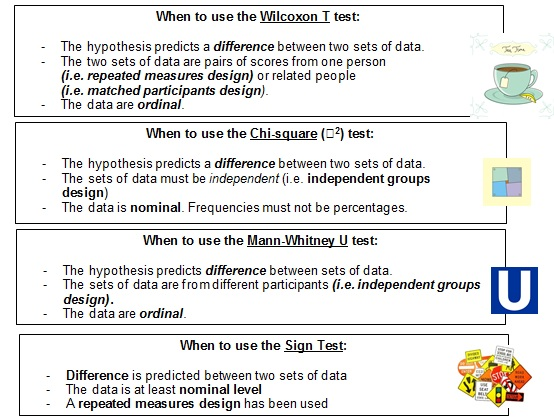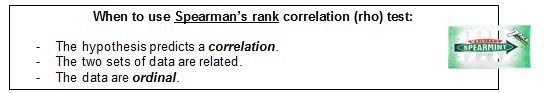Parametric Tests: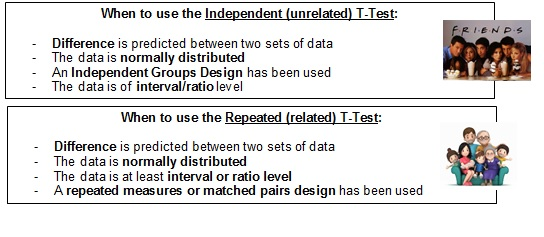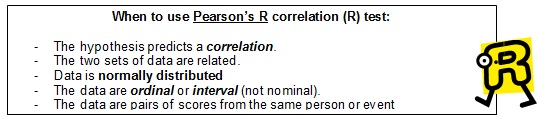A Diagram to Help: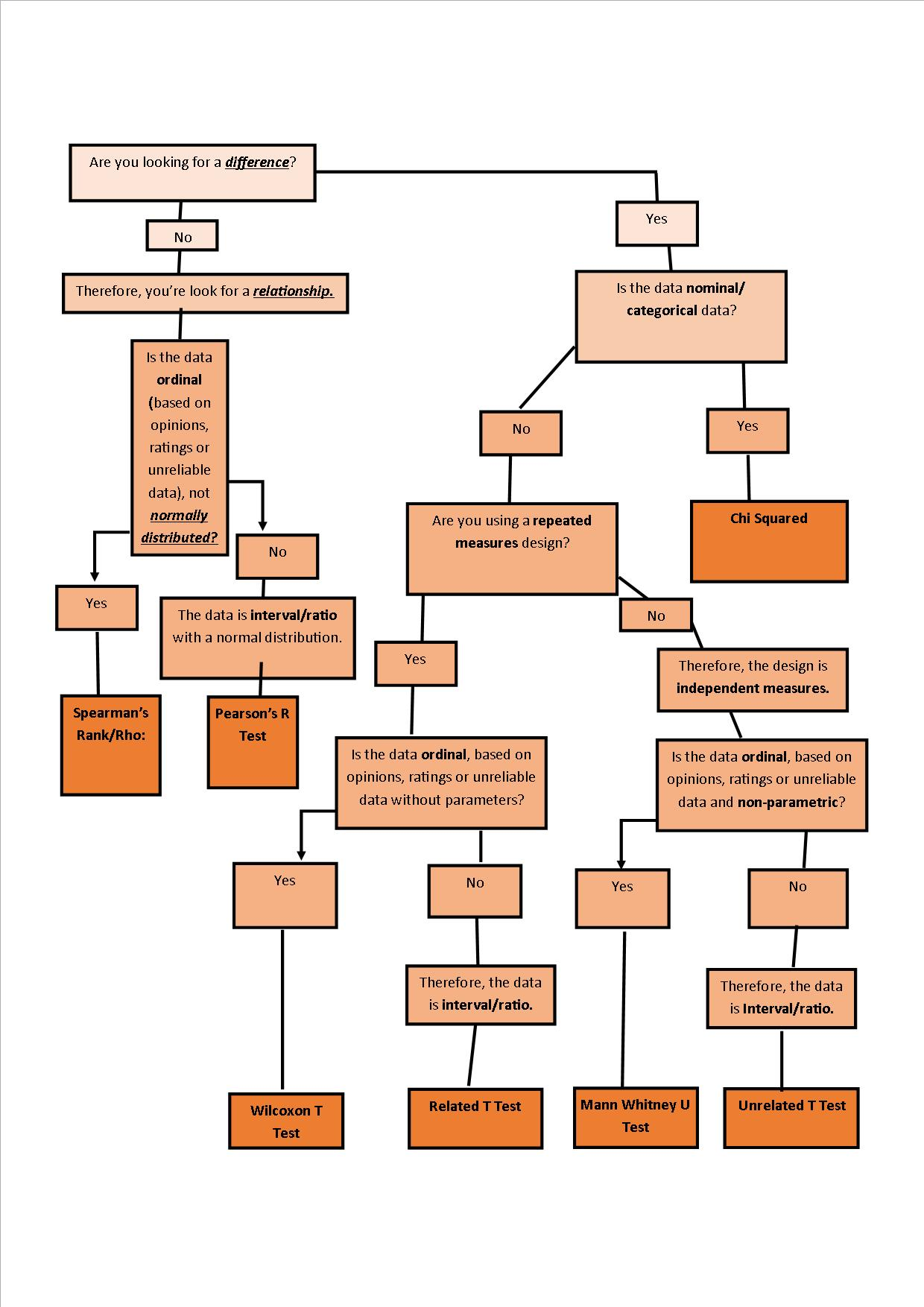### Justifying the Use of Statistical Tests

Exam Tip: This ‘justify the use of statistical test’ is a common question in the exam, you may be given a scenario/piece of research and would need to suggest what statistical test you would use (justify with the three categories that we have just mentioned above; (1) data/level of measurement, (2) experimental design, (3) stating whether the research is looking for a relationship between 2 variables or a difference).

When justifying your use of a particular statistical test, you need to explain why you chose that test. Base your answer on the experimental design and the level of data. If the question requires a third reason, add that it is a test of difference or it is a test of association (depending on whether it refers to an experiment or correlation).

For example, if you have conducted a study where you are seeking to determine whether there are differences between two independent groups of participants then you might state: In order to assess the significance of these findings it was necessary to use a statistical test. In this study an appropriate test would be a Mann-Whitney U test because (1) the design was independent measures, (2) the data was at an ordinal level and (3) a test of differences was required.

N:B You can also justify your use of statistical test by referring to parametric and non-parametric characteristics (e.g. you have used a parametric test because the data is normally distributed ans uses interval data…)

### The Use of Inferential Analysis – Observed Values and Critical values

Each inferential test (Pearson’s R, Related t-tests, Unrelated t-tests, Spearman’s Rho, Wilcoxon T, Mann-Whitney U and Chi-squared) involves taking the quantitative data collected in a study and doing some arithmetical calculations which produce a single number called the test statistic.

The Observed Value: The observed value is always the result of the statistical test. For example, the result of the Spearman’s Rho tests is the ‘rho’ value, the result of the Wilcoxon T test is the ‘T’ value, the result of the Chi Square test is the ‘x²’ value, the result of the Mann Whitney U tests is the ‘U’ value, etc…

To decide if the observed value (your observed value/result) is significant, the observed is compared to another number, called the critical value. This number is not from your research but listed in a table of critical values (you do not need to learn these tables; they will be provided in the exam). The critical value is the value that a test statistic must reach in order for the null hypothesis to be rejected. There are different tables of critical values for each inferential test. To find the appropriate critical value in a table you need to know:

1, Degrees of freedom (df) – In most cases you get this value by looking at the number of participants in the study (N). In studies using an independent groups design there are two values for N (one for each group of participants), called N1 and N2.

2, One-tailed or two-tailed test – If the hypothesis predicted at the beginning of the study was a directional hypothesis, then you use a one-tailed test, if it was non-directional then you use a two-tailed test.

3, Significance level – Selected, usually, at p ≤ 0.05, p<0.01, p<0.10

### Observed and Critical Values (and the importance of ‘R’)

Some inferential tests are significant when the observed value is equal to or exceeds the critical value, for others it is the reverse (the size of the difference between the two is irrelevant). You need to know which, and you will find it stated underneath each table. One way to remember which test requires the observed value to be higher than the critical value and which requires the opposite is by seeing if there is a letter ‘R’ in the name of the inferential test. If there is an ‘R’ (e.g. Spearman’s, Chi-square, Pearson’s R), then the observed value should be gReateR than the critical value. If there is no ‘R’ (e.g. Mann-Whitney and Wilcoxon), then the observed value should be less than the critical value.Exam Tip:  This is a Perfect Paragraph which would be useful to know for your exam when writing up whether results are significant or not).

Using the statistical test (insert the statistical test used), where the observed value of (R,T, rho etc…) is ___ (enter observed value) and the critical table value is ___ (enter critical table value) using a one/two tailed hypothesis the results can be seen to be significant/insignificant because the observed value is higher/lower than the table critical value where the P value is (enter P value) and the df are (enter df value(s) (i.e. number of participants used). As a result, the null/experimental hypothesis should be accepted and the null/experimental hypothesis rejected.

### Chi Squared and Contingency Tables

What is a contingency table? A contingency table is essentially a display format used to analyse and record the relationship between two or more variables (it is used when we have categories of data).

Chi-Square can be used to investigate “differences” (an experiment), for example, a researcher may be interested to find out whether girls or boys are more aggressive and they may study this by observing girls and boys in a nursery situation (aged 3-4). The results gained can be analysed using Chi-Square to tell us whether there is a difference between these groups

Experimental hypothesis: There will be a significant difference in the number of aggressive acts performed by boys and girls in a nursery situation.

Chi – Square – worked example for a 2×2 contingency table (using the example given above).